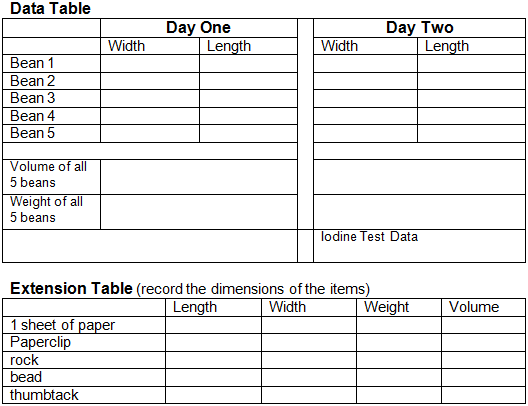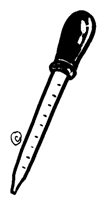Name: ____________________________________

# Measure a Bean, Why?

Overview: The purpose of this lab is for you to become familiar with the names and uses of the laboratory equipment. Two days are required to complete this experiment. All measurements and data should be placed on the attached data table.

## Day One Procedure: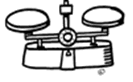1. Number 5 beans 1-5 with a pencil
2. Measure the length of and width of each bean with a ruler (use millimeters)
3. Use a beaker to fill a graduated cylinder to the 10 ml mark.
4. Add all five beans to the graduated cylinder. To determine the volume of the beans, see how many ml the water raised. (make sure to subtract the 10 ml you started with
5. Dry your beans with a paper towel. And record the weight of each bean using a scale or balance.
6. Place all five beans in a cup, write your name on the cup and fill it with 15 ml of water. (This will be stored overnight.)

Day One Questions: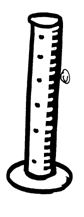1. Describe the difference between a beaker and a graduated cylinder.

2. A "unit" is a term that describes quantity. The unit of length measurements, for instance, is millimeters and centimeters. What are the units for mass (weight) and for volume? (If you didn't include these units on your table, add them in!)

3. Examine your ruler. How many millimeters are in a centimeter? _________

4. If a bean measured 2 cm, how many millimeters is it ? ___________

5. Describe the difference between mass and volume (use textbook if you need to)

6. What tool(s) is used to measure volume? _________

7. If a beaker is filled with 200 ml of water and a goldfish is added, the water level rises to 240 ml.
What is the goldfish's volume? ___________

8. If a beaker is filled with 400 ml of water and a tennis ball is added, the water level rises to 620 ml.
What is the volume of the tennis ball? _____________

## Day 2 - Procedure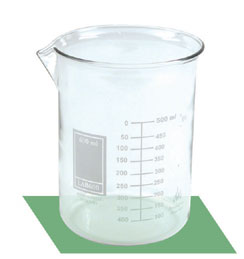1. Remove the beans from the water and record their length and widths on the data table.
2. Record the volume of the five beans
3. Record the weight of the five beans
4. Split a bean in half, and use a pipette to put a drop of iodine on the bean. Describe what happens in the table.
5. Complete the Extension Table and answer the analysis questions.

Day 2 Questions

9. Describe how the bean's width and length change after soaking.

10. Describe how the bean's weight changed after soaking.

11. Describe how the bean's volume changes after soaking.

12. How do you account for these changes?

13. Label each equipment picture printed on this lab.